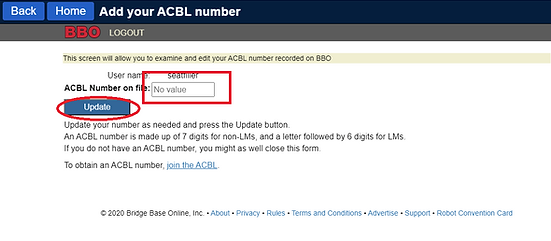# ​# ​# J=1,   K=2,   L=3,   M=4,   N=5,   O=6,    P=7,   Q=8  and  R=9)